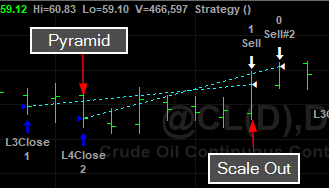If you don’t like seeing blank days in your charts then make sure you tell TradeStation to skip these Holidays.  It doesn’t make a difference in your calculations on a historic basis – TS skips these days already, but it’s more aesthetically pleasing not seeing the gaps.

Pyramiding and then Scaling Out at Different Price Levels – EasyLanguage

TOTAL, TOTAL, TOTAL – an important keyword

I just learned something new!  I guess I never programmed a strategy that pyramided at different price levels and scaled out at different price levels.Initially I thought no problem.  But I couldn’t get it to work – I tried everything and then I came across the keyword Total and then I remembered.  If you don’t specify Total in you exit directives then the entire position is liquidated.  Unless you are putting all your positions on at one time – like I did in my last post.   So remember if you are scaling out of a pyramid position use Total in your logic.

vars: maxPosSize(2);

If currentContracts < maxPosSize - 1 and c > average(c,50) and c = lowest(c,3) then buy("L3Close") 1 contract this bar on close;
If currentContracts < maxPosSize and c > average(c,50) and c = lowest(c,4) then buy("L4Close") 1 contract this bar on close;

If currentContracts = 2 and c = highest(c,5) then sell 1 contract total this bar on close;
If currentContracts = 1 and c = highest(c,10) then sell 1 contract total this bar on close;
Scaling Out Of Pyramid

Why you have to use the Total I don’t know.  You specify the number of contracts in the directive and that is sufficient if you aren’t pyramiding.  The pyramiding throws a “monkey wrench” in to the works.

First Put Multiple Contracts On:

If c > average(c,200) and c = lowest(c,3) then buy("5Large") 5 contracts this bar on close;
Using keyword contracts to put on multiple positions

Here you specify the number of contracts prior to the keyword contracts.

Easylanguage requires you to create a separate order for each exit.  Let’s say you want to get out of the 5 positions at different times and possibly prices.  Here’s how you do it:

If currentContracts = 5 and c > c then sell 1 contracts this bar on close;
If currentContracts = 4 and c > c then sell 1 contracts this bar on close;
If currentContracts = 3 and c > c then sell 1 contracts this bar on close;
If currentContracts = 2 and c > c then sell 1 contracts this bar on close;
If currentContracts = 1 and c > c then sell 1 contracts this bar on close;
One order for each independent exit

The reserved word currentContracts hold the current position size.  Intuitively this should work but it doesn’t.

{If currentContracts > 0 then sell 1 contract this bar on close;}

You also can’t put order directives in loops.  You can scale out using percentages if you like.

Value1 = 5;

If currentContracts = 5 and c > c then sell 0.2 * Value1 contracts this bar on close;
If currentContracts = 4 and c > c then sell 1 contracts this bar on close;
If currentContracts = 3 and c > c then sell 1 contracts this bar on close;
If currentContracts = 2 and c > c then sell 1 contracts this bar on close;
If currentContracts = 1 and c > c then sell 1 contracts this bar on close;
Using a percentage of original order size

That’s all there is to scaling out.  Just remember to have an independent exit order for each position you are liquidating.  You could have just two orders:  scale out of 3 and then just 2.

Setting Stop Loss and Profit Target Utilizing EntryPrice with EasyLanguage

One Problem with the “Next Bar” Paradigm – market position nor entryPrice are adjusted by the end of the bar

Whenever I develop a strategy I like to program all of my entries and exits without utilizing TradeStations built-in execution functions.  I just got use to doing this when I started programming in Fortran many years ago.  However, there a few scenarios where this isn’t possible.  If you enter a trade and use the following logic to get you out with a loss or a profit when referencing your entryPrice, you will be surprised with your results.  This is because you are telling the computer to use entryPrice before you know what it is.This logic is absolutely correct in its intention.  However, TradeStation doesn’t realize you are in a position at the end of the bar and can’t properly reference entryPrice.  Okay so we force TradeStation to only issue orders once it has a valid entryPrice.TradeStation only realizes the correct marketPosition the following day and then issues an order for the next bar.  So we get the one bar delay.  It would be helpful if TradeStation would set the marketPosition at the close of the bar on the bar of entry.   However, you can overcome this with TradeStation’s built-in execution functions.  For some reason these functions know exactly when you get in – you can also get the same results by inserting the respective strategies on the chart.

An Easy Fix ThoughBut this little bug can creep into other areas of your programming.  Keep an eye on this.